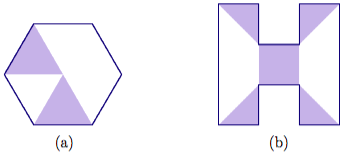# 6.10: Problem Bank

$$\newcommand{\vecs}{\overset { \rightharpoonup} {\mathbf{#1}} }$$ $$\newcommand{\vecd}{\overset{-\!-\!\rightharpoonup}{\vphantom{a}\smash {#1}}}$$$$\newcommand{\id}{\mathrm{id}}$$ $$\newcommand{\Span}{\mathrm{span}}$$ $$\newcommand{\kernel}{\mathrm{null}\,}$$ $$\newcommand{\range}{\mathrm{range}\,}$$ $$\newcommand{\RealPart}{\mathrm{Re}}$$ $$\newcommand{\ImaginaryPart}{\mathrm{Im}}$$ $$\newcommand{\Argument}{\mathrm{Arg}}$$ $$\newcommand{\norm}{\| #1 \|}$$ $$\newcommand{\inner}{\langle #1, #2 \rangle}$$ $$\newcommand{\Span}{\mathrm{span}}$$ $$\newcommand{\id}{\mathrm{id}}$$ $$\newcommand{\Span}{\mathrm{span}}$$ $$\newcommand{\kernel}{\mathrm{null}\,}$$ $$\newcommand{\range}{\mathrm{range}\,}$$ $$\newcommand{\RealPart}{\mathrm{Re}}$$ $$\newcommand{\ImaginaryPart}{\mathrm{Im}}$$ $$\newcommand{\Argument}{\mathrm{Arg}}$$ $$\newcommand{\norm}{\| #1 \|}$$ $$\newcommand{\inner}{\langle #1, #2 \rangle}$$ $$\newcommand{\Span}{\mathrm{span}}$$$$\newcommand{\AA}{\unicode[.8,0]{x212B}}$$

## Problem 28

Express the shaded portion of each figure as both a fraction and as a decimal. Justify your answers.## Problem 30

Arrange the digits 1, 2, 3, and 4 in the boxes to create the smallest possible sum. Use each digit exactly once. Justify that your answer is as small as possible.

$\frac{\Box}{\Box} + \frac{\Box}{\Box} \nonumber$

## Problem 31

Arrange the digits 1, 2, 3, and 4 in the boxes to create the smallest possible (positive) difference. Use each digit exactly once. Justify that your answer is as small as possible.

$\frac{\Box}{\Box} - \frac{\Box}{\Box} \nonumber$

## Problem 32

Use the “Dots & Boxes” model to show that $$\frac{1}{9} = 0. \bar{1}$$. Then use this fact to answer these questions and justify your answers.

1. What fraction is given by $$0. \bar{2}$$?
2. What fraction is given by $$0. \bar{5}$$?
3. What fraction is given by $$0. \bar{6}$$?
4. What fraction is given by $$0. \bar{8}$$?
5. What fraction is given by $$0. \bar{9}$$?

## Problem 33

In this problem, you will focus on the calculation

$170 \times \Box \ldotp \nonumber$

Your goal is to get a product that is close to 200.

1. Will you multiply 170 by a number greater or less than 1? Greater or less than 2? Justify your answers.
2. Suppose you can use only one decimal place. Fill in the box with a number that gets as close to 200 as possible.
3. Suppose you can use only two decimal places. Fill in the box with a number that gets as close to 200 as possible.
4. Suppose you can use only three decimal places. Fill in the box with a number that gets as close to 200 as possible.

## Problem 34

Do each computation below without using a calculator. Explain your thinking.

1. $$(23 \times 0.1) + (0.001 \times 55) \ldotp$$
2. $$18.45 \div (0.63 \div 0.7) \ldotp$$
3. $$22.65 - (0.03 \cdot 10) \ldotp$$

## Problem 35

Without actually calculating anything (just use your number sense!), order x, y, and z from smallest to largest. Explain your ordering.

$\begin{split} x &= 0.07 + 0.000001 \\ y &= 0.07 \times 0.000001 \\ z &= 0.07 \div 0.000001 \end{split} \nonumber$

## Problem 36

For each question below, choose the correct calculation and explain your choice. Then estimate the answer (don’t calculate it exactly) and explain why your estimate is a good one.

1. A large pizza has eight slices and costs \$15.95. How much does each slice of pizza cost? Should you calculate 15.95 × 8 or 15.95 ÷ 8?
2. There are 2.54 centimeters in an inch. A standard sheet of notebook paper is $$8 \frac{1}{2}$$ inches wide and 11 inches long. How many centimeters wide is the page? Should you calculate 8.5 × 2.54 or 11 × 2.54 or 8.5 ÷ 2.54 or 11 ÷ 2.54?
3. In a model train set, 1.38 inches represents one foot in real life. The height of One World Trade Center in New York City is 1776 feet. How tall would a scale model of the building be? Should you calculate 1776 × 1.38 or 1776 ÷ 1.38?
4. Eight-tenths of a jumprope is 1.75 meters long. How long is the whole rope? Should you calculate 0.8 × 1.75 or 0.8 ÷ 1.75 or 1.75 ÷ 0.8?

## Problem 37

Kaimi had no money at all when he cashed his paycheck. As he left the bank, he bought a piece of candy for a nickel from a machine. Later, he realized that the money in his pocket was equal to twice his paycheck. After a quick calculation, he figured out what happened: the teller accidentally switched the dollars and cents. How much was Kaimi supposed to be paid, and what did the teller give him? Justify your answer.

## Problem 38

Here are the rules to a card game. Read the rules carefully and then answer the questions below.

• Each player starts with 10 points. The goal is to score as close to 100 points as possible without going over.
• On your turn: draw two cards, which will each have a decimal number on them. Using estimation (no computation), you can choose to multiply or divide your current score by one of the decimal numbers.
• After you decide, compute your new score exactly using a calculator. If your new score is over 100, you lose. If not, the other player takes a turn.
• At the end of your turn, you can decide to end the game. If you do, the other player gets one more turn. Then, the player with the score that is closest to 100 without going over wins the game.

Here are the questions:

1. On your turn, your score is 50. You draw the cards 0.2 and 1.75. Remember that your choices are: $$\begin{split} &\text{divide by}\; 0.2 \qquad \text{multiply by}\; 0.2 \\ &\text{divide by}\; 1.75 \qquad \text{multiply by}\; 1.75 \ldotp \end{split}$$What is your best move and why?
2. On your turn, your score is 88. You draw 1.3 and 0.6. What is your best move and why?
3. Your partner has a score of 57, and your score is 89. On her turn, your partner draws 0.8 and 1.8. She says she wants to end the game. On your final turn, you draw 0.7 and 1.2. If you both make the best possible move, who will win the game? Justify your answer.

This page titled 6.10: Problem Bank is shared under a CC BY-SA 4.0 license and was authored, remixed, and/or curated by Michelle Manes via source content that was edited to the style and standards of the LibreTexts platform; a detailed edit history is available upon request.